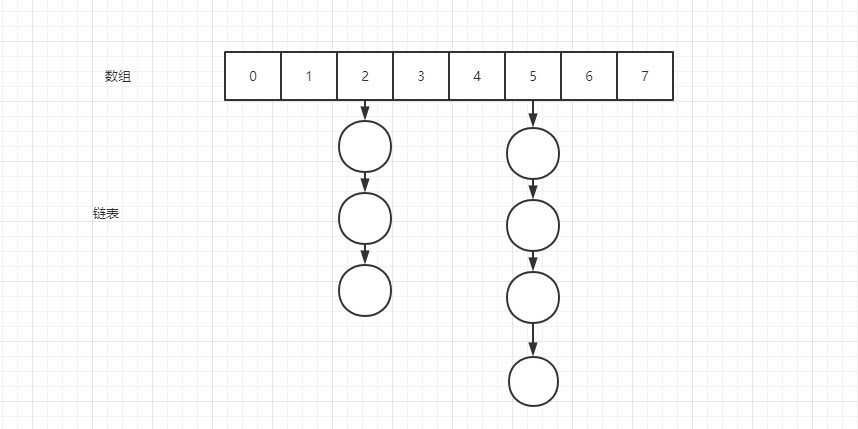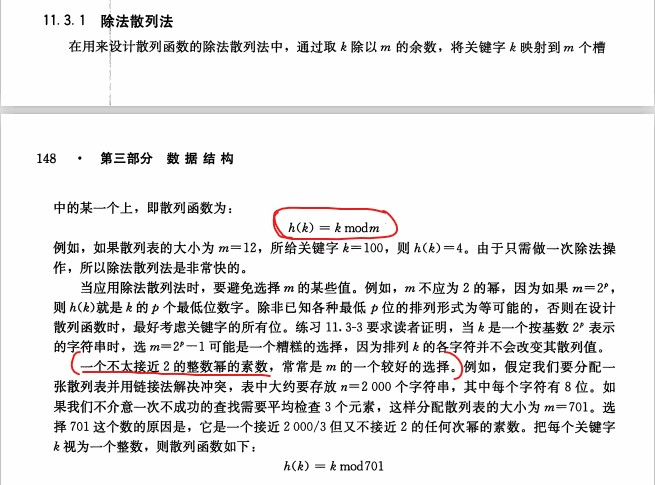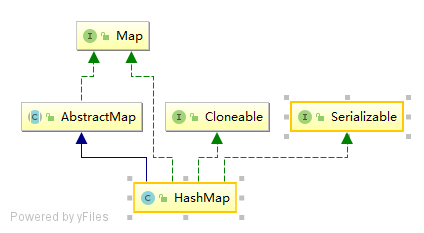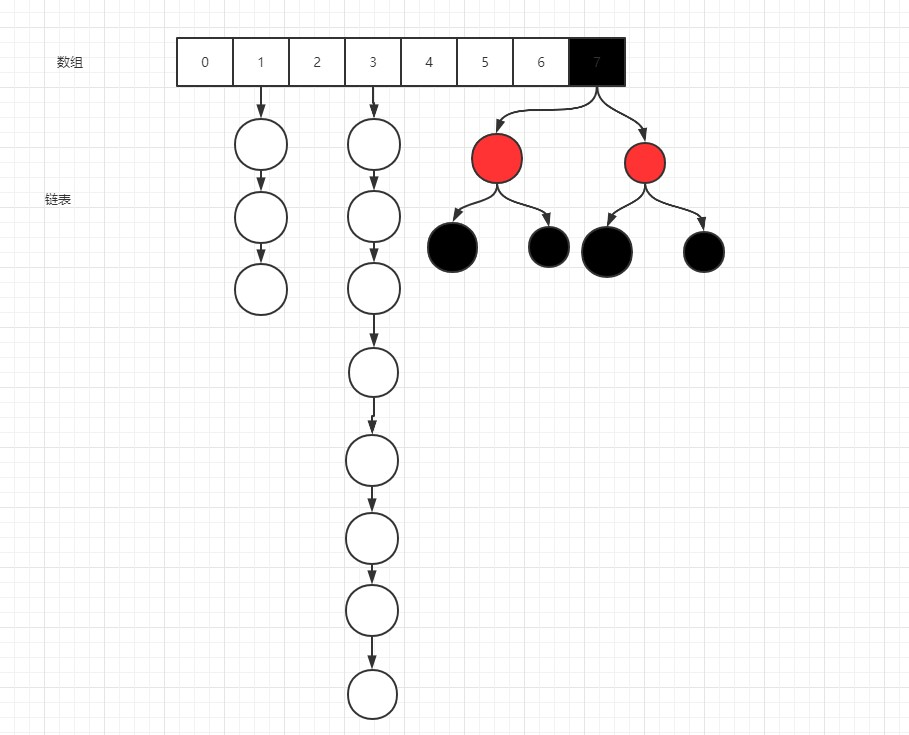# 前言

• ArrayList

• List存储数据是单一元素，而Map存储数据是K-V形式存储的

## 为什么要有K,V形式来存储数据？

• Session
• Redis
• HBase
• ...

• HashMap
• TreeMap

HashMap很长，文采略烂，大家要有耐心哦

# 哈希表

## 什么是哈希表？

PS：概念都不是人话，不用记他，直接看结构# HashMap## 操作方法

### 属性值介绍

``````Map<String, String> hashMap = new HashMap<>();
Map<String, String> hashMap = new HashMap<>(20);``````

``````/**MUST be a power of two.*/
// 默认初始化长度
static final int DEFAULT_INITIAL_CAPACITY = 1 << 4;
// 负载因子，负责判定数据存储到什么地步的时候进行扩容
static final float DEFAULT_LOAD_FACTOR = 0.75f;

public HashMap() {
}``````

``````public HashMap(int initialCapacity) {
}

public HashMap(int initialCapacity, float loadFactor) {
if (initialCapacity < 0)
throw new IllegalArgumentException("Illegal initial capacity: " +
initialCapacity);
if (initialCapacity > MAXIMUM_CAPACITY)
initialCapacity = MAXIMUM_CAPACITY;
throw new IllegalArgumentException("Illegal load factor: " +
this.threshold = tableSizeFor(initialCapacity);
}

// 除外，传递参数不同，
public HashMap(Map<? extends K, ? extends V> m) {
putMapEntries(m, false);
}``````

Hashtable中，是按照除法散列法中的规范来做的：也就是上面说的不太接近2的整数幂的素数，但是为什么在HashMap中就没有采用这种规范，而是要采用2的N次幂呢？我们后面再具体说

``````static final int tableSizeFor(int cap) {
int n = cap - 1;
n |= n >>> 1;
n |= n >>> 2;
n |= n >>> 4;
n |= n >>> 8;
n |= n >>> 16;
return (n < 0) ? 1 : (n >= MAXIMUM_CAPACITY) ? MAXIMUM_CAPACITY : n + 1;
}``````

``````static final int TREEIFY_THRESHOLD = 8;
static final int UNTREEIFY_THRESHOLD = 6;``````

• 也就是说，在JDK1.8中，HashMap的底层结构采用的是哈希表+红黑树的形式``````/*
* the frequency of
* nodes in bins follows a Poisson distribution
* (http://en.wikipedia.org/wiki/Poisson_distribution) with a
* parameter of about 0.5 on average for the default resizing
* threshold of 0.75, although with a large variance because of
* resizing granularity. Ignoring variance, the expected
* occurrences of list size k are (exp(-0.5) * pow(0.5, k) /
* factorial(k)). The first values are:
*
* 0:    0.60653066
* 1:    0.30326533
* 2:    0.07581633
* 3:    0.01263606
* 4:    0.00157952
* 5:    0.00015795
* 6:    0.00001316
* 7:    0.00000094
* 8:    0.00000006
* more: less than 1 in ten million
*
*/``````

### 方法说明

• Node
``````static class Node<K,V> implements Map.Entry<K,V> {
final int hash;
final K key;
V value;
Node<K,V> next;

Node(int hash, K key, V value, Node<K,V> next) {
this.hash = hash;
this.key = key;
this.value = value;
this.next = next;
}
// ...
}``````

• TreeNode
``````static final class TreeNode<K,V> extends LinkedHashMap.Entry<K,V> {
TreeNode<K,V> parent;  // red-black tree links
TreeNode<K,V> left;
TreeNode<K,V> right;
TreeNode<K,V> prev;    // needed to unlink next upon deletion
boolean red;
TreeNode(int hash, K key, V val, Node<K,V> next) {
super(hash, key, val, next);
}
//...
}``````

#### put

``````hashMap.put("key1", "value1");

public V put(K key, V value) {
return putVal(hash(key), key, value, false, true);
}``````

``````static final int hash(Object key) {
int h;
return (key == null) ? 0 : (h = key.hashCode()) ^ (h >>> 16);
}``````

``````final V putVal(int hash, K key, V value, boolean onlyIfAbsent,
boolean evict) {
Node<K,V>[] tab; Node<K,V> p; int n, i;
if ((tab = table) == null || (n = tab.length) == 0)
n = (tab = resize()).length;                            // 注释1
if ((p = tab[i = (n - 1) & hash]) == null)
tab[i] = newNode(hash, key, value, null);               // 注释2
else {
Node<K,V> e; K k;
if (p.hash == hash &&
((k = p.key) == key || (key != null && key.equals(k))))     // 注释4
e = p;
else if (p instanceof TreeNode)
e = ((TreeNode<K,V>)p).putTreeVal(this, tab, hash, key, value);// 注释5.2
else {
for (int binCount = 0; ; ++binCount) {              // 链表的插入过程
if ((e = p.next) == null) {
p.next = newNode(hash, key, value, null);
if (binCount >= TREEIFY_THRESHOLD - 1)              // 注释5
treeifyBin(tab, hash);
break;
}
if (e.hash == hash &&
((k = e.key) == key || (key != null && key.equals(k))))
break;
p = e;
}
}
if (e != null) {                                    // 注释4.2
V oldValue = e.value;
if (!onlyIfAbsent || oldValue == null)
e.value = value;
afterNodeAccess(e);
return oldValue;
}
}
++modCount;
if (++size > threshold)                                 // 注释3
resize();
afterNodeInsertion(evict);
return null;
}``````

##### 计算索引下标

``````static int indexFor(int h, int length) {
return h & (length - 1);
}``````

`JDK1.8`中：

``i = (n - 1) & hash``

(n-1)之后的二进制： 01111

hash = 18，转成二进制： 10010

&运算之后： 00010

``````hashMap.put("key1", "value1");
hashMap.put("key1", "value2");
System.out.println(hashMap);

// {key1=value2}``````
##### 转红黑树存储数据

``````final void treeifyBin(Node<K,V>[] tab, int hash) {
int n, index; Node<K,V> e;
if (tab == null || (n = tab.length) < MIN_TREEIFY_CAPACITY)
resize();
else if ((e = tab[index = (n - 1) & hash]) != null) {
TreeNode<K,V> hd = null, tl = null;
do {
TreeNode<K,V> p = replacementTreeNode(e, null);
if (tl == null)
hd = p;
else {
p.prev = tl;
tl.next = p;
}
tl = p;
} while ((e = e.next) != null);
if ((tab[index] = hd) != null)
hd.treeify(tab);
}
}``````

#### resize()

``````final Node<K,V>[] resize() {
Node<K,V>[] oldTab = table;
int oldCap = (oldTab == null) ? 0 : oldTab.length;              // oldCap = 16
int oldThr = threshold;                                         // oldThr = 12
int newCap, newThr = 0;
if (oldCap > 0) {
if (oldCap >= MAXIMUM_CAPACITY) {
threshold = Integer.MAX_VALUE;
return oldTab;
}
else if ((newCap = oldCap << 1) < MAXIMUM_CAPACITY &&       // newCap = 32
oldCap >= DEFAULT_INITIAL_CAPACITY)
newThr = oldThr << 1; // double threshold               // newThr = 24
}
else if (oldThr > 0) // initial capacity was placed in threshold
newCap = oldThr;
else {               // zero initial threshold signifies using defaults
newCap = DEFAULT_INITIAL_CAPACITY;
}
if (newThr == 0) {
float ft = (float)newCap * loadFactor;
newThr = (newCap < MAXIMUM_CAPACITY && ft < (float)MAXIMUM_CAPACITY ?
(int)ft : Integer.MAX_VALUE);
}
threshold = newThr;
@SuppressWarnings({"rawtypes","unchecked"})
Node<K,V>[] newTab = (Node<K,V>[])new Node[newCap];
table = newTab;
if (oldTab != null) {
for (int j = 0; j < oldCap; ++j) {
Node<K,V> e;
if ((e = oldTab[j]) != null) {
oldTab[j] = null;
if (e.next == null)
newTab[e.hash & (newCap - 1)] = e;
else if (e instanceof TreeNode)
((TreeNode<K,V>)e).split(this, newTab, j, oldCap);
else { // preserve order
Node<K,V> loHead = null, loTail = null;
Node<K,V> hiHead = null, hiTail = null;
Node<K,V> next;
do {
next = e.next;
if ((e.hash & oldCap) == 0) {
if (loTail == null)
else
loTail.next = e;
loTail = e;
}
else {
if (hiTail == null)
else
hiTail.next = e;
hiTail = e;
}
} while ((e = next) != null);
if (loTail != null) {
loTail.next = null;
}
if (hiTail != null) {
hiTail.next = null;
}
}
}
}
}
return newTab;
}``````

• 容器长度的扩容是成倍扩容的
• 判断容器扩容的依据，也就是说在初始通过（容器长度 * 负载因子）得到的扩容依据的数也会成倍增长的，所以这里我们要注意一下
##### 数据迁移

`JDK1.8`中数据迁移是判断：当前hash & 旧容器长度的结果：

• 如果结果是0，在新数组中还是在老位置
• 如果结果不是0，那么在新数组中的位置为：原数组中的位置 + 原数组的容器长度

``````// e.hash = 65 通过计算为0，
if ((e.hash & oldCap) == 0) {
if (loTail == null)
else
loTail.next = e;
loTail = e;
}
// e.hash = 6366 通过计算不为0，走else
else {
if (hiTail == null)
else
hiTail.next = e;
hiTail = e;
}

// 原数组中的位置和新数组中位置相同
if (loTail != null) {
loTail.next = null;
}
// 新数组中的位置改变
if (hiTail != null) {
hiTail.next = null;
}``````

HashMap关键点也就聊完了，下面我们来总结一下

# 总结

• HashMap底层采用哈希表+红黑树的结构来存储，无序存储。当哈希表链表长度>8的时候会将链表转成红黑树，判断基准是通过采用泊松分布经过验证之后得出的结论；然后在当红黑树的节点<6的时候将红黑树的结构转回到链表（官方没有明确表示为什么是6）
• HashMap在默认情况下容器长度为：16，且如果需要扩容的话，那么会扩容当前容器长度的2倍。且达到扩容的条件时当前容器长度*负载因子，在后续扩容过程中，扩容条件为成倍扩容
• 这里我们在构造的时候也可以传入固定参数，但是这里需要注意：如果需要自定义容器长度的话，最好定义的长度是2的N次幂：
• 因为在通过key的哈希值查找数组索引的时候会采用`&`计算，性能对比`%`更高
• 同时在数据迁移的过程中，判断key的哈希值与原数组长度通过运算之后是否为0，等于0的话就是在新数组中的下标位置不变，否则的话就等于将当前下标位置 + 原数组的长度即在新数组中的索引位置
• HashMap是线程不安全的类，如果想要保证线程安全的话：
• 自己加锁
• Collections.synchronizedMap()
• 采用ConcurrentHashMap

HashMapAPI文档